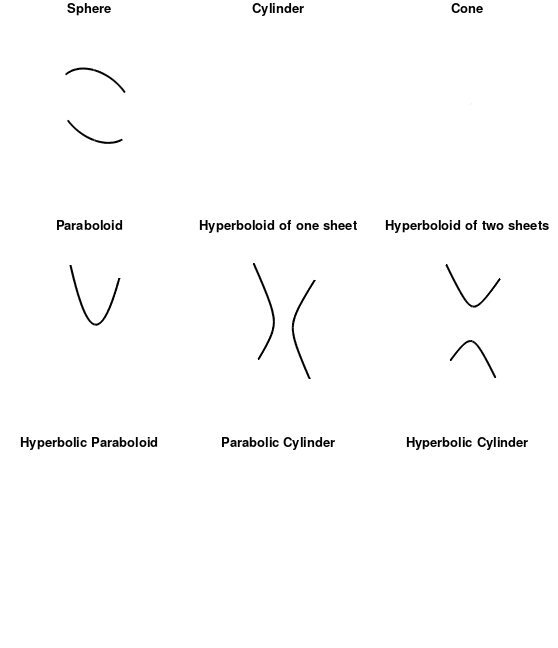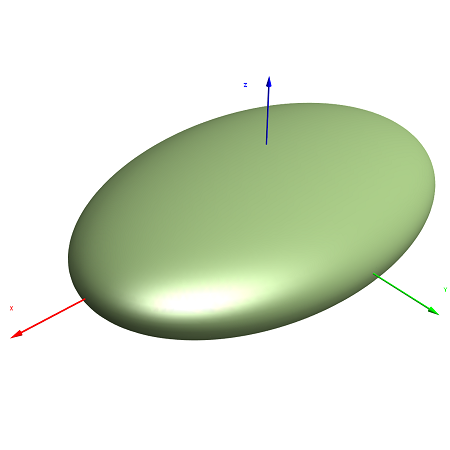A quadric surface is a 3D extension of a conic (ellipsis, hyperbola, or parabola). In 2D space, they are defined by quadratic equations in 2D space . Quadric refers to the degree of the equation describing the surface: variables in these equations are raised to the 2nd power. They can be described, in general, as a graph of an equation expressed in the form :

Ax2 + B2 + C2 + Dxy + Exz + Fyz + Hx + Iy + Jz + K = 0.

Where A, B, C, D, E, F, H, I, J, K are fixed constants and x, y, z, are variables.

Different types of quadric surfaces can be obtained from simplifying this equation by rotations and translations of the x, y, z coordinate axes.There are six basic types of quadric surface :

1. Ellipsoid,
2. Elliptic paraboloids,
3. Hyperbolic paraboloid,
4. Cones,
5. Hyperboloids of one sheet,
6. Hyperboloids of two sheets.

Other examples of quadratic surfaces include the cylinder, elliptic cone, elliptic cylinder, elliptic hyperboloid, hyperbolic cylinder, paraboloid, sphere, and spheroid.

1. EllipsoidThe general equation for an ellipsoid is:If A = B = C, the shape is a sphere.

2. Elliptic paraboloidsThe equation for an elliptic paraboloid is:Elliptic paraboloids have ellipses as cross sections. If A = B, the cross section is a circle.

3. Hyperbolic paraboloid

The equation for a hyperbolic paraboloid is:Hyperbolic paraboloids are saddle-shaped, like a Pringle. The sign of c determines whether the graph opens up or down. A positive value for c has the saddle right side up (as if it were being placed on a horse).

4. Cones
The equation for a cone is :5. Hyperboloids of one sheet
The equation for the hyperboloid of one sheet is:6. Hyperboloids of two sheets
The equation for the hyperboloid of two sheets is: